# Analytical Reasoning - Online Test

Q1. Find the number of triangles in the given figure.Explaination / Solution:

The figure may be labelled as shown.The simplest triangles are AHG, AIG, AIB, JFE, CJE and CED i.e. 6 in number.

The triangles composed of two components each are ABG, CFE, ACJ and EGI i.e. 4 in number.

The triangles composed of three components each are ACE, AGE and CFD i.e. 3 in number.

There is only one triangle i.e. AHE composed of four components.

Therefore, There are 6 + 4 + 3 + 1 = 14 triangles in the given figure.

Q2. Find the minimum number of straight lines required to make the given figure.Explaination / Solution:

The figure may be labelled as shown.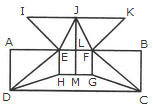The horizontal lines are IK, AB, HG and DC i.e. 4 in number.

The vertical lines are AD, EH, JM, FG and BC i.e. 5 in number.

The slanting lines are IE, JE, JF, KF, DE, DH, FC and GC i.e. 8 is number.

Thus, there are 4 + 5 + 8 = 17 straight lines in the figure.

Q3. Find the number of triangles in the given figure.Explaination / Solution:

The figure may be labelled as shown.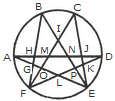The simplest triangles are AGH, GFO, LFO, DJK, EKP, PEL and IMN i.e. 7 in number.

The triangles having two components each are GFL, KEL, AMO, NDP, BHN, CMJ, NEJ and HFM i.e. 8 in number.

The triangles having three components each are IOE, IFP, BIF and CEI i.e. 4 in number.

The triangles having four components each are ANE and DMF i.e. 2 in number.

The triangles having five components each are FCK, BGE and ADL i.e. 3 in number.

The triangles having six components each are BPF, COE, DHF and AJE i.e. 4 in number.

Total number of triangles in the figure = 7 + 8 + 4 + 2 + 3 + 4 = 28.

Q4. Find the number of triangles in the given figure.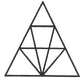Explaination / Solution:

The figure may be labelled as shown.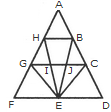The simplest triangles are AHB, GHI, BJC, GFE, GIE, IJE, CEJ and CDE i.e. 8 in number.

The triangles composed of two components each are HEG, BEC, HBE, JGE and ICE i.e. 5 in number.

The triangles composed of three components each are FHE, GCE and BED i.e. 3 in number.

There is only one triangle i.e. AGC composed of four components.

There is only one triangle i.e. AFD composed of nine components.

Thus, there are 8 + 5 + 3 + 1 + 1 = 18 triangles in the given figure.

Q5. Find the number of triangles in the given figure.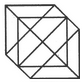Explaination / Solution:

The figure may be labelled as shown.The simplest triangles are IJO, BCJ, CDK, KQL, MLQ, GFM, GHN and NIO i.e. 8 in number.

The triangles composed of two components each are ABO, AHO, NIJ, IGP, ICP, DEQ, FEQ, KLM, LCP and LGP i.e.10 in number.

The triangles composed of four components each are HAB, DEF, LGI, GIC, ICL and GLC i.e. 6 in number.

Total number of triangles in the figure = 8 + 10 + 6 = 24.

Q6. Find the minimum number of straight lines required to make the given figure.Explaination / Solution:

The figure may be labelled as shown.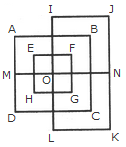The horizontal lines are IJ, AB, EF, MN, HG, DC and LK i.e. 7 in number.

The vertical lines are AD, EH, IL, FG, BC and JK i.e. 6 in number.

Thus, there are 7 + 6 = 13 straight lines in the figure.

Q7. Find the number of triangles in the given figure.Explaination / Solution:

The figure may be labelled as shown.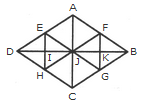The simplest triangles are AFJ, FJK, FKB, BKG, JKG, JGC, HJC, HIJ, DIH, DEI, EIJ and AEJ i.e. 12 in number.

The triangles composed of two components each are JFB, FBG, BJG, JFG, DEJ, EJH, DJH and DEH i.e. 8 in number.

The triangles composed of three components each are AJB, JBC, DJC and ADJ i.e. 4 in number.

The triangles composed of six components each are DAB, ABC, BCD and ADC i.e. 4 in number.

Thus, there are 12 + 8 + 4 + 4 = 28 triangles in the figure.

Q8. Find the number of triangles in the given figure.Explaination / Solution:

The figure may be labelled as shown.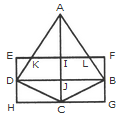The simplest triangles are AKI, AIL, EKD, LFB, DJC, BJC, DHC and BCG i.e. 8 in number.

The triangles composed of two components each are AKL, ADJ, AJB and DBC i.e. 4 in number.

The triangles composed of the three components each are ADC and ABC i.e. 2 in number.

There is only one triangle i.e. ADB composed of four components.

Thus, there are 8+ 4 + 2 + 1= 15 triangles in the figure.

Q9. Find the number of triangles in the given figure.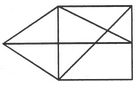Explaination / Solution:

The figure may be labelled as shown.The simplest triangles are ABF, BIC, CIH, GIH, FGE and AFE i.e. 6 in number.

The triangles composed of two components each are ABE, AGE, BHF, BCH, CGH and BIE i.e. 6 in number.

The triangles composed of three components each are ABH, BCE and CDE i.e. 3 in number.

Hence, the total number of triangles in the figure = 6 + 6 + 3 = 15.

Q10. Find the number of triangles in the given figure.Explaination / Solution:

The figure may be labelled as shown.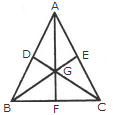The simplest triangles are AGE, EGC, GFC, BGF, DGB and ADG i.e. 6 in number.

The triangles composed of two components each are AGC, BGC and ABG i.e. 3 in number.

The triangles composed of three components each are AFC, BEC, BDC, ABF, ABE and DAC i.e. 6 in number.

There is only one triangle i.e. ABC composed of six components.

Thus, there are 6 + 3 + 6 + 1 = 16 triangles in the given figure.